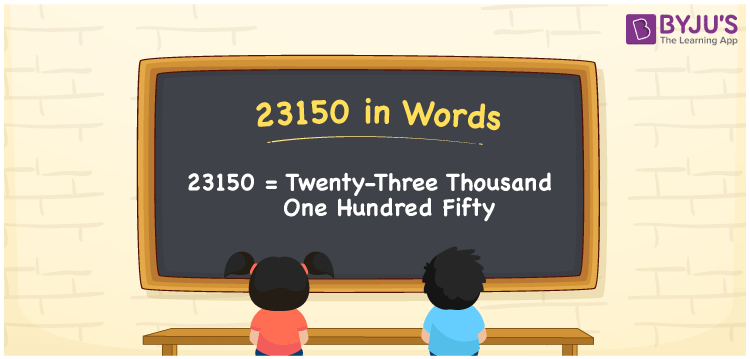# 23150 in Words

23150 in words is written as Twenty-Three Thousand One Hundred Fifty. For example, Rohan saved Rs. 23150 in three months, then you can say, “Rohan saved Rupees Twenty-Three Thousand One Hundred Fifty in three months”. By using a place value of a specific number, we can convert the number to words easily. Hence, a place value table is essential to create a numerical name. 23150 is a cardinal number as it shows a certain amount. In this article, let us learn how to express the number 23150 in words.

 23150 in Words Twenty-Three Thousand One Hundred Fifty Twenty-Three Thousand One Hundred Fifty in numerical form 23150

## 23150 in English Words

English is the most widely used language in the education field. Hence, it is necessary for students to learn numbers in words using the English alphabet. Hence, we can spell the number 23150 in English as Twenty-Three Thousand One Hundred Fifty.## How to Write 23150 in Words?

Conversion of numbers to words is very easy with the help of a place value chart. The table provided below represents the place value chart for the number 23150.

 Ten-Thousands Thousands Hundreds Tens Ones 2 3 1 5 0

Therefore, we can write the expanded form as:

2 x 10000 + 3 x 1000 + 1 x 100 + 5 x 10 + 0 x 1

= 20000 + 3000 + 100 + 50 + 0

= 20000 + 3000 + 100 + 50

= 23150

= Twenty-Three Thousand One Hundred Fifty

Hence, 23150 in words is written as Twenty-Three Thousand One Hundred Fifty

Interesting way of writing 23150 in words

2 = Two

23 = Twenty-Three

231 = Two Hundred and Thirty-One

2315 = Two Thousand Three Hundred Fifteen

23150 = Twenty-Three Thousand One Hundred Fifty

Thus, the word form of the number 23150 is Twenty-Three Thousand One Hundred Fifty

23150 is a natural number that precedes 23151 and succeeds 23149

• 23150 in words – Twenty-Three Thousand One Hundred Fifty
• Is 23150 an odd number? – No
• Is 23150 an even number? – Yes
• Is 23150 a perfect square number? – No
• Is 23150 a perfect cube number? – No
• Is 23150 a prime number? – No
• Is 23150 a composite number? – Yes

## Frequently Asked Questions on 23150 in Words

Q1

### How to write 23150 in words?

23150 in words is written as Twenty-Three Thousand One Hundred Fifty.
Q2

### Simplify 21000 + 2150, and express in words.

21000 + 2150 = 23150. Hence, 23150 in words is Twenty-Three Thousand One Hundred Fifty.
Q3

### Convert Twenty-Three Thousand One Hundred Fifty into numbers.

Twenty-Three Thousand One Hundred Fifty in numbers is 23150.